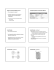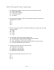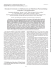# December 17, 2012 Naming Covalent Compounds What is a covalent compound again?

## Transcription

December 17, 2012 Naming Covalent Compounds What is a covalent compound again?
```December 17, 2012
Naming Covalent Compounds
What is a covalent compound again?
A compound composed of two or more elements that are bonded together because of sharing
electrons.
➡ Nonmetal and a nonmetal
****Naming covalent compounds is completely different than naming of the ionic compounds*****
Rules:
1.) Use Prefixes to tell how many of each element. The second element ends in -ide.
Example 1: N O Dinitrogen pentoxide
Example: N Cl
Dinitrogen tetrachloride
2.) If the first element listed has just one, it does not get a prefix:
A.) NO Nitrogen dioxide
B.) CO Carbon Dioxide
3.) The second element always gets a prefix no matter
what:
A.) CO
Carbon Monoxide
B.) NO
Nitrogen Monoxide
4.)Cannot reduce covalent compound formulas.
A.) N O will not be reduced to NO
They are completely different compounds.
Practice: Write the names of the following.
1.) SO
2.) ICl
3.) PBr
Write the formulas for the following:
4.) carbon tetrachloride.
Date: December 18, 2012
Bell Work:
1.) Li P
2.) N O
3.) CSe
4.) SIyt
Naming Ionic Compounds
What are the names of the ions?
Naming Captions (+ charge)
K name of element
Potassium Cation or Potassium Ion
Ca Calcium Cation or Calcium Ion
Naming Anions (- charge)
Cl name of element ending in "ide" Anion
Chloride Anion
Or Chloride Ion
O Oxide Anion or Oxide ion
We know how to get the oxidation number from the periodic table for the main group of
elements.
Group # 1, 2, 13, 14, 15, 16, 17, 18
+1, +2, +3, +/-4, -3, -2, -1, none
What are the oxidation numbers listed for the elements not listed (groups 3->12 "transition
metals"
In the periodic table given to you look at the key for oxidation states, find the element and the ion
could be a couple of choices.
Example: Iron (Fe) could be a +2, +3, or +6.
In the periodic table given to you look at the Key for oxidation states, find the element and the ion could be a
couple of choices.
Ex. Iron (Fe) could be +2, +3, or +6
We use Roman numerals to differentiate from the different ions.
Fe Iron (II) ion
Fe Iron (III) ion
Fe Iron (VI) ion
Roman Numerals:
Number:
1, 2, 3, 4, 5, 6, 7
Roman Numeral: I, II, III, IV, V, VI, VII
Naming Ionic Compounds : NO PREFIXES!!!!!!!
NaCl Sodium Chloride
BaCl Barium Chloride
K O. Potassium Chloride
January 2, 2013
Bell Ringer:
Write formulas for....
1.) Silver Bromide
2.) Chromium III Oxide
3.) Silicon Dioxide
Reviewing Homework: Transition Metals
2.) Titanium II Oxide
3.) Titanium III Oxide
4.) Titanium IV Oxide
5.) Tin II Oxide
Notes:
Polyatomic Ions
1.) Write the formula Tin (IV) Sulfate
The 2 on the outside of the parenthesis means that there are two sulfate groups (so technically 2 S and 8
O.
2.) Strontium Carbonite
Sr(CO2) would be understood to have a one outside the parenthesis, and since it is a one, we can drop
the parenthesis.
3.) Write the name for the formula for the ionic compound. RuPO
The one tripled up going to the phosphate ion +3, so the superscript of the one phosphate must also triple going
up to the ruthenium ion, so the charge in doubt is +3.
Ruthenium III phosphate
1.) Sodium Carbonate
2.) Copper II Sulfate
3.) KClO
4.) NH OH
5.) Fe(ClO )
January 3, 2013
Bell Ringer: Name Mn(CrO )
1.) Silver Hydroxide
2.) Magnesium Nitrate
3.) Magnesium Sulfate
4.) Iron III Sulfate
5.) Platinum IV Phosphate
6.) Ammonium Hydroxide
7.) NaNO
8.) NH Cl
9.) BeCO
10.) Ca(ClO )
11.) Fe(BrO )
Mixed Review:
1.) NiCl
2.) Na O
3.) SeO
5.) RuCO
6.) Silver Oxide
7.) Dinitrogen Pentoxide
8.) Chromium (III) Phosphate
9.) Copper (II) Oxide
4.) NaClO
10.) Aluminum Bromide
January 4, 2013
1.) SF
2.) Na S
Review Homework from Yesterday.
Write a paragraph about the way we name compounds.
In order to properly name compounds you first need to determine which elements that you are working with. You will
need to check to see if these elements are both nonmetals or a metal and a nonmetal.
If they are two nonmetals, then you know that the chemical bond will be a COVALENT BOND. With covalent bonds,
we always will name the elements based on how many of element there are in the compound. To name it if it has more
than one of the same element you will give it a prefix, such as di-, tri-, quad-, or penta-. However the starting element if
it has just one you will not use a prefix.
If you have a metal and a nonmetal, the bond will be IONIC. In ionic bonds, there are NO PREFIXES! Metals always go
before the nonmetal. You will check to see the ions first and write them down. Then you will cross the element charges
from one element to the other and write them as a subscript. For Polyatomic Ions, use the Polyatomic sheets to help you
with the charges and then follow the same procedure for ionic compounds.
Acids An acid is a distinct type of molecular compound. Usually refers to a
solution in water of one of these special compounds rather than to the
compound itself. Binary acids are acids that consists of two elements, usually hydrogen
and one of the halogens - F, Cl, Br, and I. Oxyacids are acids that contain of hydrogen, oxygen, and a third
element (usually a nonmetal) Most acids are based in water and have very particular properties .
Hydrofluoric acid
Hydrochloride acids
Nitric acids (Nitrate)
Sulfuric acid (Sulfate)
Phosphoric acid (Phosphate)
Acetic Acid (acetate)
Carbonic Acid (carbonate)
January 7, 2013
Bell Ringer: Name the following.
a.) CS
b.) AgNO
c.) VCO
Practice Sheet #1-36
1.) LiCl
2.) KOH
5.) FeCl
9.) Al (SO )
3.) HgOH
4.) kCl
6.) LiNO
7.) NH OH
8.) Cu O
10.) N O
11.) NaOH
12.) CO
13.) CoO
14.) Pb(OH)
15.) NH NO
16.) NA CO
17.) HgO
18.) Zn(NO )
19.) Cu PO
20.) CsOH
21.) Li O
22.) Ca(OH)
23.) CaBr
25.) Cu SO
26.) FeCO
27.) SO
29.) Al(OH)
30.) NaClO
31.) NaIO
24.) Fe O
28.) Ba(BrO )
32.) Na SO
January 9, 2013
Bell ringer: What percentage of this class is male? *grab a
calculator*
Review of formula writing from
yesterday.
Percent Composition - percent by mass of each element in a compound.
What is the percent composition of H O?
Percentage Paragraph:
In order to find the Percent Composition of a compound you will first need to find the
value of the atomic weights of each element in the compound. Once you have the atomic
weights, you will need to multiply the atomic weight by how many of each element is in the
compound. This will give you the atomic weight of the number of that particular element.
Then you need to add the weights of each element in the compound together to find the
total mass (atomic weight). Once you have the total you are going to want to find the part
of each element out of the entire total which will look like a fraction. You want to solve
the fraction and get a decimal. Once you have a decimal, you will want to multiply it by
100 to get a percentage. Do that for each element.
January 10, 2013
Bell Ringer: what's the difference between molecular formula and empirical formula?
Molecular Formula - a chemical formula that shows the number and kinds of atoms
in a molecule, but not the arrangement of the atoms. Example: C H O
Empirical Formula - a chemical formula that shows the composition of a compound
in terms of the relative numbers and kinds of atoms in the simplest ratio.
Example C H O -> C H O
How to Find the Empirical Formula:
pg 247 #3 CaBr 20.0 compound
1.) A compound is found to contain 63.52% iron and 36.48% sulfur. Find it's
empirical formula.
Paragraph on Finding Empirical Formulas. First you find the percentage of the element
in the compound if not already given to you. Then you would divide the percentage of
the element into its atomic weight (which you would find on the periodic table of
elements). Then look to see which quotient of each element is the smallest. Divide that
January 11, 2013
What is the empirical formula for C H ?
Practice 1&2 on page 249.
1.) Determine the molecular formula of the compound with an empirical formula of CH
and a formula mass of 78.110 amu.
2.) A sample of a compound with a formula mass of 34.00 amu is found to consist
of 0.44g H and 6.92 O. Find it's molecular formula.
Write a paragraph.
In order to find the molecular formula from the empirical formula
Pg. 249 #4
If 4.04g of N combine with 11.46g O to produce a compound with a formula mass
of 108.0 amu, what is the molecular formula of this compound?
```

### Completing Chemical Reactions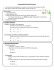### Chapter 8 Ionic Compounds: Names and Formulas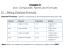### AP WORKSHEET 0: Significant Figures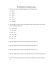### Regents chemistry textbook page 28 #1-14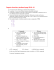### Compound V\lords Compound VVords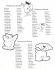### Simple and Compound Sentences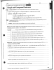### 16 Naming Ionic Compounds-S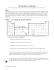### Covalent Formulas and Nomenclature Say Thanks to the Authors (No sign in required)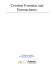### Untitled - IISER Pune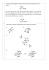### 1. A problem is listed below. Identify its type.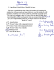### Rukan Brochure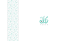### How to Figure Out Chemical Formulas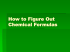### Modifying the Compound Rest on the 7x10 Mini Lathe For Quick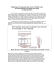### pdf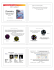### Find the structure for the following molecules: Problem 1.### Document 6608629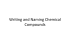### Document 6546924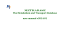### CHAPTER 2 ATOMS, MOLECULES, AND IONS Table 1. Key terms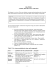### Why Do Chemical Bonds Form? Comparing Ionic and Covalent Bonds